# Infinitesimal deformation

infinitesimally-small deformation

A concept which first appeared in the description of the deformation of a surfacein three-dimensional Euclidean space, in which the variation of the lengths of curves onis of a lower order of magnitude than the change in the spatial distance between the points of these curves. In fact, the theory of infinitesimal deformations deals with vector fields and quantities associated with them, defined at the points ofand satisfying equations which represent the linearizations of the deformation equations of.

Thus, if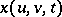is the position vector of a deformation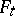of the surface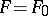, an infinitesimal deformation ofis characterized by the (initial) deformation rate, i.e. by the vector field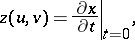which satisfies the equation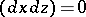or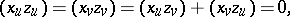(1)

whereis the position vector of. The vector fieldis also known as the velocity field of the infinitesimal deformation or as the bending field. A vectorcan be uniquely defined such that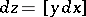. The set of points of the space described by the position vectoris called the rotation diagram of the infinitesimal deformation. See also Darboux surfaces.

In a more general situation, the infinitesimal deformation of a manifoldimbedded in a Riemannian space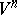represents an isometric variation of the imbedding, i.e. such a vector field along the imbeddingwhere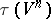is the tangent bundle to, which satisfies the equation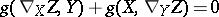(1prm)

on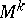; here,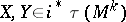are vector fields tangent to the imbedding,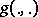is the Riemannian metric ofand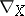is the covariant derivative with respect to the Levi-Civita connection oncorresponding to. The field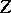uniquely determines the field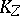of anti-symmetric tensors of type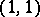along the imbedding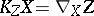, satisfying the equation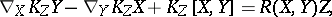whereis the Riemannian curvature operator of.

Ifis induced by a Killing vector field, i.e.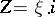, then the corresponding infinitesimal deformations (and alsoitself) are called trivial. Ifallows only trivial infinitesimal deformations, then it is called rigid. (Cf. Rigidity.)

Under a geodesic mapping, an infinitesimal deformation ofwith a vector fielduniquely corresponds to an infinitesimal deformation of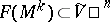with the vector field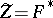, and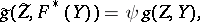whereis the potential of the mapping. In particular, this correspondence exists under a projective transformation of the Euclidean space (the Darboux–Sauer theorem) and under a geodesic mapping of the Euclidean space into a space of constant curvature (a Pogorelov transformation).

To isometric variations of higher orders correspond infinitesimal deformations of higher orders; unlike for the first-order infinitesimal deformations discussed above, only isolated results, mainly concerning surfaces of rotation, are available.

The theory of infinitesimal deformations has numerous applications in mathematics and mechanics. Principal applications include problems of isometric imbedding by the method of extension along a parameter, studies of isometric surfaces in spaces of constant curvature (cf. Cohn-Vossen transformation), in problems of rigidity of shells, etc.